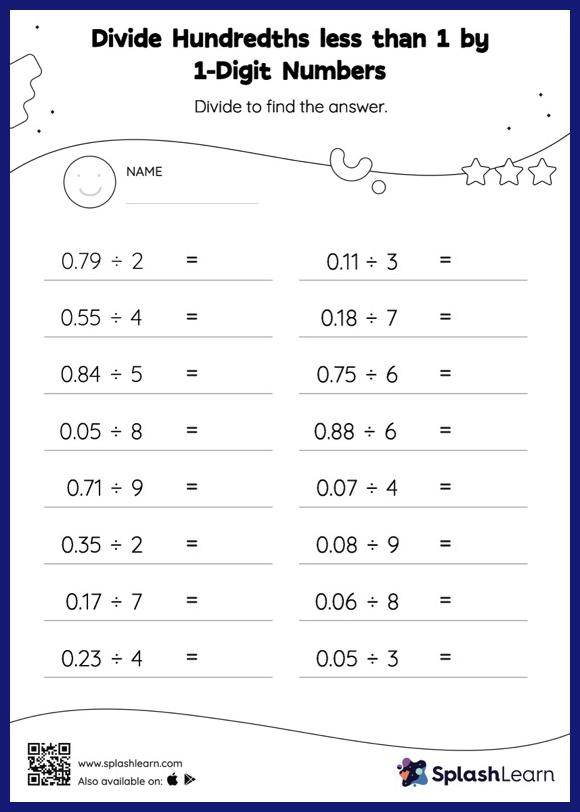# Divide Hundredths less than 1 by 1-Digit Numbers with Remainder: Horizontal Division Worksheet

Home > Divide Hundredths less than 1 by 1-Digit Numbers with Remainder: Horizontal DivisionStudents divide decimals by 1-digit numbers in the same manner as they would divide whole numbers first. Depending on how many decimal digits are in the dividend, they add a decimal point at the appropriate place in the quotient. Their accuracy with this concept is improved by using the divide hundredths less than 1 by 1-digit numbers with remainder worksheet. As the worksheet uses a horizontal format, it allows for more creativity when it comes to how the student solves the problem. In contrast, in the vertical format, students usually employ the standard method to solve.